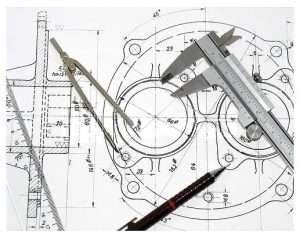• TRUE RADIUS means that the arc is part of a circle. The height must be less than or equal to half the width of the unit.
• HALF CIRCLE means that the height is exactly half the width. A true half circle is a true radius, but a true radius but a true radius does not have to be a true half circle. (i.e. Eyebrow)
• EYEBROW is a true radius but not a true half circle. (See drawing to right)
• ELLIPSE is a pointed arch, especially one having only two centers and equal radii.
• GOTHIC means that the height must be greater than half the width.
• SPRING LINE is the point where radius or curved portion meets straight vertical legs.
• CENTER LINE is the measurement from the bottom of the unit to the top of the radius.
• DROP is the measurement from the top of the unit to the spring line.